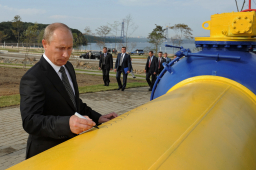# Gas prices 2021

According to the SPP price list in 2021, the price of natural gas, together with its supply, is as follows:

D1 - we cook. Fix 3.34 euros/month and 0.0479 euros/kWh (1 cubic meter of gas is approximately 10.555 kWh. It is called combustion heat and changes over time as the ratio of natural gas components in wells/wells changes).

D2 - heating. The fixed is EUR 6.91/month, and the variable component is EUR 0.0355/kWh.

A. Calculate the limit value of consumption in kWh, at which the price for gas supply is the same in rates D1 and D2. For example, below this consumption, it pays to be D1. If it is higher, the rate D2 pays off.

B. Since the gas meter measures the volume of natural gas consumed in cubic meters, convert this value to m3 as well.

C. Calculate how many times electricity is more expensive than energy in gas.
at the rate (tariff) DD1 costs 2.47 Eur + 0.1766 Eur/kWh
at the rate (tariff) DD2 costs 6.36 Euros + 0.138 Euros/kWh

The example arose in the autumn of 2021 in response to the sharp rise in gas prices (300-700%) on the spot market due to its shortage and the pressure of money by the central bank of the ECB, the Fed, and the suspicion of a harsh winter. Other factors are the shift from dirtier coal to electricity generation from gas, the closure of atoms, and steady wind - low wind farm production.

x =  287.9032 kWh
x2 =  27.2765 kWh
c =  3.7871

### Step-by-step explanation:Did you find an error or inaccuracy? Feel free to write us. Thank you!

Tips for related online calculators
Looking for help with calculating arithmetic mean?
Looking for a statistical calculator?
Do you have a linear equation or system of equations and looking for its solution? Or do you have a quadratic equation?

#### Grade of the word problem:

We encourage you to watch this tutorial video on this math problem: